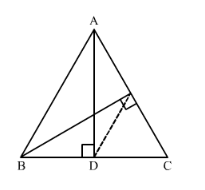# In ∆ABC, AD and BE are altitude. Prove that

Question:

In $\triangle \mathrm{ABC}, \mathrm{AD}$ and $\mathrm{BE}$ are altitude. Prove that $\frac{\operatorname{ar}(\Delta \mathrm{DEC})}{\operatorname{ar}(\Delta \mathrm{ABC})}=\frac{\mathrm{DC}^{2}}{\mathrm{AC}^{2}}$.

Solution:Given: ΔABC in which AD and BE are altitudes on sides BC and AC respectively.

Since ADB = AEB = 90°, there must be a circle passing through point D and E having AB as diameter.

We also know that, angle in a semi-circle is a right angle.

Now, join DE.

So, ABDE is a cyclic quadrilateral with AB being the diameter of the circle.

∠A + BDE = 180° [Opposite angles in a cyclic quadrilateral are supplementary]

⇒ ∠A + (BDA + ADE) = 180°

⇒ ∠BDA + ADE = 180° − A ..... (1)

Again,

∠BDA + ADC = 180° [Linear pair]

⇒ ∠BDA + ADE + EDC = 180°

⇒ ∠BDA + ADE = 180° − EDC ..... (2)

Equating (1) and (2), we get

180° − A = 180° − EDC

⇒ ∠A = EDC

Similarly, B = CED

Now, in ΔABC and ΔDEC, we have

∠A = EDC

∠B = CED

∠C = C

∴ ΔABC  ΔDEC

$\Rightarrow \frac{\text { Area of } \triangle \mathrm{DEC}}{\text { Area of } \triangle \mathrm{ABC}}=\left(\frac{\mathrm{DC}}{\mathrm{AC}}\right)^{2}$